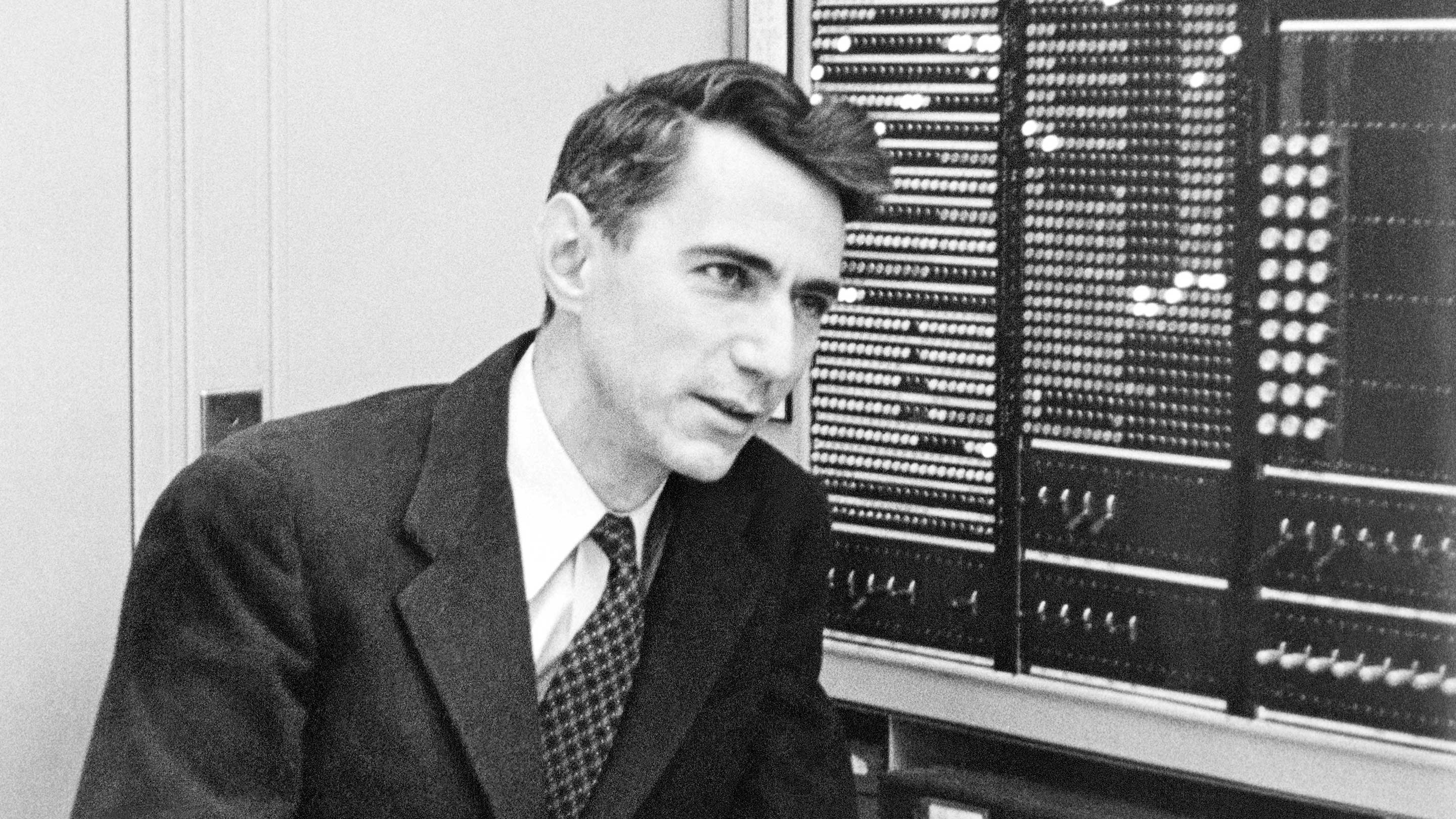## Information EntropyEntropy is a measure of uncertainty of a random variable's possible outcomes.

It's highest when there are many equally likely outcomes. As you introduce more predictability (one of the possible values of a variable has a higher probability), Entropy decreases.

It measures how many "questions" on average you need to guess a value from the distribution. Since you'd start by asking the question that is most likely to get the correct answer, distributions with low Entropy would require smaller message sizes on average to send.

The entropy of a variable from distribution $p$ is expressed as: $H=\sum\limits_{i=1}^{n} p_{i} \times log_2(\frac{1}{p})$

The expression is commonly inverted and rewritten like this: $H=-\sum\limits_{i=1}^{n} p_{i} \times log_2(p_i)$

When using log base 2, the unit of Entropy is a bit (a yes or no question).

In code:

Claude Shannon borrowed the term Entropy from thermodynamics as part of his theory of communication.